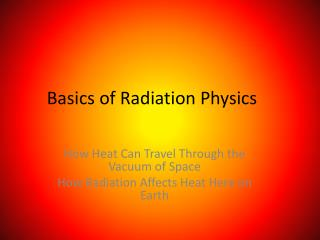DownloadDownload PresentationDownload Presentation- - - - - - - - - - - - - - - - - - - - - - - - - - - E N D - - - - - - - - - - - - - - - - - - - - - - - - - - -
##### Presentation Transcript

1. Basics of Radiation Physics How Heat Can Travel Through the Vacuum of Space How Radiation Affects Heat Here on Earth

2. EM Spectrum

3. Wavelength vs. Frequency λν = c Lambda*nu = C wavelength*frequency = speed of light C = 3.00 x 108 m/s (in a vacuum)

4. Example 1 λν = c Frequency of green light? Wavelength 500 nm = 5 x 10-7 m ν = c/ λ= (3x108 m/s)/(5 x 10-7m) = 6 x1014 s-1 1 s-1 = 1/(2π) Hertz (cycles/sec) ω = 3800 GHz

5. Example 2 λν= c Wavelength of FM radio waves? Frequency 103 MHz 1 s-1 = 1/(2π) Hertz λ = c/ν = (3x108 m/s)/(1.6x107 s-1) = 18.3 meters (!)

6. Planck Relation E = hν E = energy of one photon h = Planck Constant = 6.63 x 10-34 J s Can also write: E = hc/λ

7. Example: Energy of Green Photons E = hc/λ E = (6.6 x 10-34 J s)(3 x108 m/s) 500 x 10-9 m = 4.0 x 10-9 J/photon x 6.02 x 1023 photons/mole = 2.4 x 105 J = 240 J/mole

8. Compare to Carbon Fixation CO2 + H2O -> Glucose G = +120 kJ/mole Light has energy of ~200 kJ/mole Can visible light provide enough energy to fix carbon?

9. Stefan-Boltzmann Law